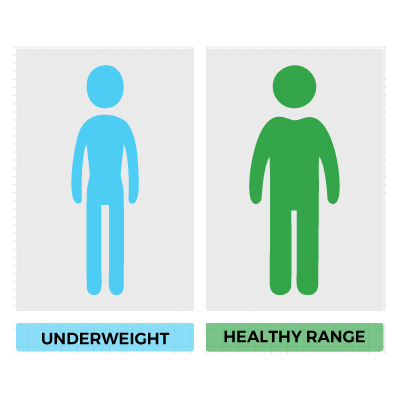﻿ BMI Calculator | Check & Calculate Body Mass Index Online

# BMI Calculator

#### Check and Calculate Body Mass Index

An online BMI calculator tool with metric, imperial formula system. It check, measure & calculate body mass index online. Check BMI percentile score for adults and kids.

## BMI Chart and Categories

 Very Severely Underweight Less than 15 Severely Underweight Between 15 - 16 Underweight Between 16 - 18.5 Normal Weight Between 18.5 - 24.9 Overweight Between 25 - 29.9 Moderately Obese Between 30 - 35 Severely Obese Between 35 - 40 Very Severely Obese 40 or greater

### BMI Formula

Calculating BMI using the Metric System - Metric BMI Formula

BMI=mass (kg) / height2 (m)

With the metric system, the formula for BMI is weight in kilograms divided by height in meters squared. Since height is commonly measured in centimeters, an alternate calculation formula, dividing the weight in kilograms by the height in centimeters squared, and then multiplying the result by 10,000, can be used.

BMI Imperial Formula

When using English measurements or Imperial system, ounces (oz) and fractions must be changed to decimal values. Then, calculate BMI by dividing weight in pounds (lbs) by height in inches (in) squared and multiplying by a conversion factor of 703.

BMI=703 × mass (lbs) / height2(in)

#### BMI Calculation for Kids, Children and Teens:

For children, BMI differs by age and gender. BMI is used as a screening tool to identify possible weight problems for children. For children and teens, BMI is age- and gender-specific and is often referred to as BMI-for-age. BMI is calculated using the same method as adults, but is expressed, not as an absolute value, but as a percentile which can be obtained from either a graph or a percentile calculator. These percentiles express a child’s BMI relative to other children of the same gender and age.

#### Weight Status BMI Percentiles Score for Children and Teens

Underweight: Less than the 5th percentile

Healthy weight: 5th percentile to less than the 85th percentile

Overweight 85th to less than the 95th percentile

Obese: Equal to or greater than the 95th percentile

#### What are the limitations of BMI?

The clinical limitations of BMI should be considered. BMI is a surrogate measure of body fatness because it is ameasure of excess weight rather than excess body fat. Factors suchas age, sex, ethnicity, and muscle mass can influence therelationship between BMI and body fat.### Spreading Knowledge Across the World

USA - United States of America  Canada  United Kingdom  Australia  New Zealand  South America  Brazil  Portugal  Netherland  South Africa  Ethiopia  Zambia  Singapore  Malaysia  India  China  UAE - Saudi Arabia  Qatar  Oman  Kuwait  Bahrain  Dubai  Israil  England  Scotland  Norway  Ireland  Denmark  France  Spain  Poland  and  many more....